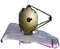# NIRISS Point Spread FunctionsThe simulated JWST NIRISS imaging point spread functions (PSFs) in each filter and predicted values for the full width at half maximum (FWHM), radial profiles, and encircled energy curves can be found using WebbPSF.

On this page

Predicted point spread functions (PSFs) for every JWST instrument can be simulated using WebbPSF. Given a wavefront error budget for the mirror alignment, WebbPSF can create multiple realizations of the PSF with a given filter. Each of these realizations assumes various contributions to the wavefront error from several optical components, which cause the PSF shape and orientation to vary slightly.

WebbPSF allows the user to select between a "predicted" optical path difference (OPD) map and a slightly more conservative "requirements" OPD map. The following figures show one realization of PSFs for NIRISS imaging in each filter assuming the "requirements" OPD map. Below is some introductory information used to calculate these PSFs.

At wavelengths λ > 2 μm, JWST obtains diffraction-limited imaging with a Strehl ratio = 0.8 and PSF full width at half maximum (FWHM) of ~λ/D radians (JWST's D = 6.5 m mirror). The NIRISS detector achieves Nyquist sampling or better (FWHM > 2 pixels) above ~4 µm. Below these wavelengths, the PSF is under-sampled. PSF sampling may be improved by performing subpixel dithers between exposures.

# Simulated NIRISS PSFs

The PSFs were made with a wavelength sampling of 20 wavelengths per filter and a pixel over-sampling of 9 in order to achieve FWHM and encircled energy values to be accurate to 1%. Both the detector and over-sampled PSFs are centered on the pixel (rather than on the pixel corner).

The PSFs with filters in the longer wavelength range, specifically filters F227W - F480M, use the CLEARP aperture which has an occultation in the form of a pupil alignment reference (PAR). The shape of this PAR can be seen in Figures 1 through 3 on the NIRISS Pupil and Filter Wheels page. The reduced transmission from the CLEARP aperture has been compensated for by normalizing the PSFs to the OPD's exit pupil (rather than the entrance pupil). However, the presence of the PAR does cause morphological differences in the PSFs created with these longer wavelength filters.

Figure 1 shows the detector-sampled PSF with each NIRISS filter on a log scale. Figure 2 zooms in to the cores of the PSFs.NIRISS PSFs with each filter were simulated by WebbPSF. Each PSF image is 77 × 77 detector pixels (~5.05" on a side). All are displayed with the same log scale. PSFs are shown in the detector pixel scale (0.0656”). Note that the the morphological differences in the PSFs made with the long-wave filters can be explained by the occultations of the PAR in the CLEARP filter.These are zooms into the central 15.24 × 15.24 pixels (1" on a side) of each simulated PSF shown in Figure 1. Each PSF is centered within a pixel and displayed with the same log scaling as Figure 1.

# PSF FWHM

Figure 3 shows the FWHM as a function of the filter’s average wavelength for each simulated PSF. Numerical values for each FWHM, in units of arcsec and pixels, are reported in Table 1. These values were calculated with WebbPSF v 0.7.0 using the PSF parameters described in the previous section. Note: due to severe under-sampling below ~4 µm, the over-sampled PSFs were used to calculate the FWHM.This figure shows the FWHM measured for each of the over-sampled PSFs simulated by WebbPSF, shown in Figures 1 and 2. Each point, color-coded by wavelength, corresponds to one of the 12 filters in Table 1. Note: the longer wavelength filters are used with the CLEARP aperture which has a central obstruction and therefore these PSFs follow a different diffraction limit.

Table 1. FWHM values (in arcsec and pixels) for each PSF, from Figure 3

FilterWavelength (μm)PSF FWHM (arcsec)PSF FWHM (pixel)
F090W0.8980.0420.643
F115W1.1440.0410.627
F140M1.4030.0480.732
F150W1.4890.0500.770
F158M1.5830.0530.814
F200W1.9670.0650.991
F277W2.7440.0861.318
F356W3.5530.1111.699
F380M3.8260.1211.847
F430M4.2840.1362.067
F444W4.3810.1372.089
F480M4.8140.1522.319

# Radial Profiles

Figure 4 shows the radial profiles for each simulated, over-sampled PSF. The radial profiles have been normalized to the value of the peak pixels.In these plots of the radial profiles for each simulated PSF, the pixel size (0.0656") is reported in each panel as a dotted line. Note that the the morphological differences in the radial profiles of the PSFs made with the long-wave filters can be explained by the occultations of the PAR in the CLEARP filter.

# Encircled Energy

Figure 5 shows the encircled energy curves for each simulated, over-sampled PSF. Numerical values for 50% and 80% encircled energy (the fraction of light contained in a circular aperture) for each simulated PSF, extracted from the center of the pixel, are shown in Figure 5.

The curves are normalized to the exit pupil in order to account for the CLEARP aperture blocking some of the light on longer wavelength filters.In these encircled energy curves for each PSF, the radius (in arcsec and pixels) at which the encircled energy is 50% and 80% is reported for each simulated PSF, centered on the pixel.

Table 2 lists the average encircled energy radii, calculated from a grid of WebbPSF (v. 0.7.0) models. The grid has 121 positions within the pixel, with 0.1 pixel spacing from the edge to the center of the pixel, and 10 realizations per position per filter.

Table 2.  Radius (in arcsec and pixels) at which the encircled energy (EE) is 50% and 80%

Filterradius(EE=50%) (arcsec)

radius(EE=50%)(pixel)

radius(EE=80%) (arcsec)radius(EE=80%)(pixel)
F090W0.081.22

0.22

3.35
F115W0.081.18

0.20

3.06
F140M0.081.18

0.19

2.83
F150W0.081.19

0.18

2.80
F158M0.081.20

0.18

2.77
F200W0.081.26

0.18

2.79
F277W0.111.68

0.33

5.01
F356W0.121.89

0.38

5.72
F380M0.131.97

0.39

6.00
F430M0.142.10

0.41

6.28
F444W0.142.13

0.42

6.37
F480M0.152.31

0.44

6.71

Published 13 Nov 2018 11 Dec 2019 Updated to use average encircled energy radii based on grid of WebbPSF values for Table 2